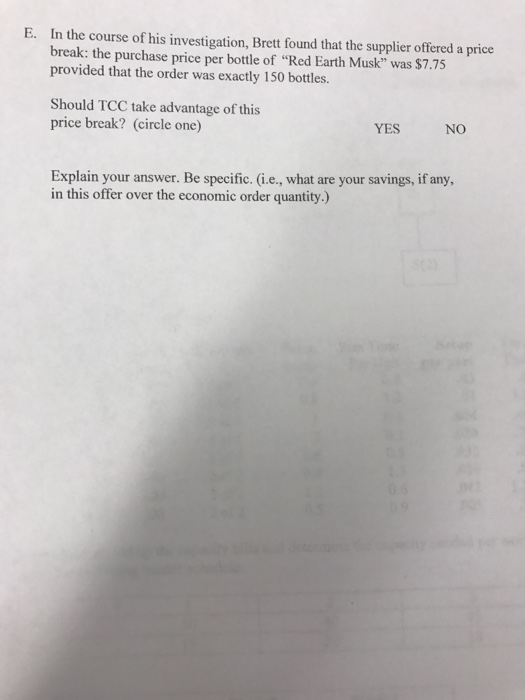# Solved: Please provide me with an ELIGIBLE and UNDERSTANDABLE answer, thank youWe have following information

Weekly demand = 35 bottles per week

Don't use plagiarized sources. Get Your Custom Essay on
Solved: Please provide me with an ELIGIBLE and UNDERSTANDABLE answer, thank you
GET AN ESSAY WRITTEN FOR YOU FROM AS LOW AS \$13/PAGE

Cost of placing an order S = \$ 2.50 per order

Holding or carrying cost H = \$0.75 per bottle per year

The purchase price P = \$8 per bottle

Number of operating weeks in a year = 48 weeks, therefore 48 orders per year

Therefore annual demand D = 35 bottles *48 weeks = 1680 bottles

A. Weekly order size = 35 bottles per week

Total annual cost = Total ordering cost + total holding cost + Total purchase Cost

Where,

Total ordering cost = Cost of placing an order * number of orders per year = \$ 2.50 *48 = \$120

Total holding cost = average inventory * Holding cost per bottle per year = (Weekly order size/2)* \$0.75

= (35/2) * \$0.75 = \$13.125

Total purchase Cost = purchase price * annual demand = \$ 8 * 1680 bottles = \$13,440

Therefore,

Total annual cost = \$120 +\$13.125 + \$13,440 = \$13,573.125

B. For minimum cost, the order quantity should be Economic Order Quantity (Q). Formula for Economic order quantity

Q = √ (2 * Annual Demand * cost of placing order/ carrying cost)

OR Q = √ (2* D*S/H)

= √ (2*1680*\$2.50/\$0.75) = 105.83 bottles or 106 bottles (nearest whole number)

C. Safety stock = z * St. Dev. Of demand * sqrt (L)

Where,

The z value for the desired Service Level of 95% = 1.65 (given)

Standard deviation of demand = 7 bottles

L is the lead time = 2 weeks

Let’s calculate the safety stock at 95% service level

Safety stock = 1.65 * 7 * sqrt (2) = 16.33 bottles

D. Periodic inventory control system for TCC by using z = 2.05.

We can calculate reorder point in following manner

Reorder Point = average weekly demand * lead time + safety stock

OR,

Reorder Point Q = D *L + z * St. Dev. Of demand * sqrt (L)

= 35 * 2 + 2.05 *7 *√2 = 90.29 or 90 bottles (nearest whole number)

In Periodic inventory control system for TCC, the company should place an order when in hand inventory is approx. 90 bottles

E. Economic Order Quantity Q = 105.83 or 106 bottles

And

Total Annual cost = total ordering cost + total carrying cost + purchase cost

Total Annual Inventory cost = (D/Q)* S + (H*Q)/2 + \$8 * 1680

= (1680/105.83) * \$2.50 + (\$0.75 * 105.83)/2+ \$8 * 1680 = \$13,519.37

If order quantity Q = 150 and purchase cost = \$7.75 per bottle

Total Annual cost = total ordering cost + total carrying cost + purchase cost

Total Annual Inventory cost = (D/Q)* S + (H*Q)/2 + \$7.75 * 1680

= (1680/150) * \$2.50 + (\$0.75 * 150)/2 + \$7.75 * 1680= \$13,104.25

Yes, TCC should take advantage of this price break as the total annual cost is less for order size of 150.